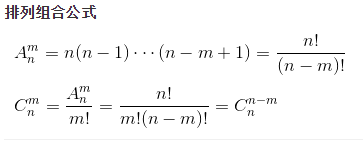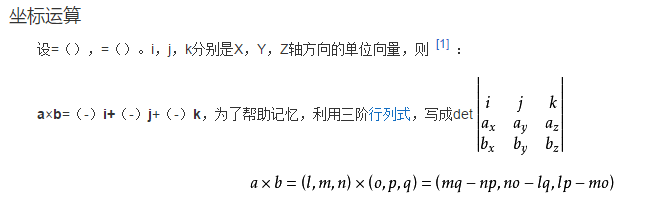• 向量叉积求平面平行四边形面积
千次阅读
2021-05-31 20:38:17

向量OA（x1,y1）,OB(x2,y2)double最多输出12位

平面叉积坐标表达式：S=x1*y2-x2*y1;要取绝对值哦；

若为平行四边形：y1=k1*x1+b1;y2=k1*x2+b2;y3=k2*x3+b3;y4=k2*x4+b4;

则：S=（b4-b3）*(b2-b1)/(k1-k2);注意也要取绝对值；

例题：

#include<stdio.h>
#include<math.h>
int main(){
double k1,k2,b1,b2,b3,b4,price,s,ans;
int T;
scanf("%d",&T);
while(T--){
scanf("%lf%lf%lf%lf%lf%lf%lf",&k1,&k2,&b1,&b2,&b3,&b4,&price);
s=(b4-b3)*(b2-b1)/(k1-k2);
ans=s*price;
ans=fabs(ans);
printf("%.10lf\n",ans);
}
return 0;
} 

更多相关内容
•文档
•文档
• struct Point{//两个点相减是向量,二维向量叉乘是平行四边形面积 int x,y; Point(int _x = 0, int _y = 0) :x(_x), y(_y) {} Point operator -(const Point &amp;p)const { return Point(x - p.x, y - p.y)...struct Point{//两个点相减是向量,二维向量叉乘是平行四边形面积
int x,y;
Point(int _x = 0, int _y = 0) :x(_x), y(_y) {}
Point operator -(const Point &p)const {
return Point(x - p.x, y - p.y);
}
long long operator ^(const Point &p) const {
return (long long)x*p.y - (long long)y*p.x;
}
}p[maxn];
面积 = abs((p[k] - p[i]) ^ (p[j] - p[i]));其实只要把二维向量看做第三维数值为0的三维空间向量就行了， 根据三维向量行列式运算的结果，所得向量只有第三维非0，也就是说，V1(x1, y1) X V2(x2, y2) = x1y2 – y1x2，展开全文• 平行四边形的周长公式平行四边形的周长等于(长边(a)+短边(b))乘以2。计算公式L=2*(a+b) ，其中a表示长边，b表示短边，L表示周长，如图所示：如图所示如果知道a,h,和角度α也可以计算出平行四边行的周长，图中b=h/sin...

平行四边形的周长公式

平行四边形的周长等于(长边(a)+短边(b))乘以2。计算公式L=2*(a+b) ，其中a表示长边，b表示短边，L表示周长，如图所示：如图所示如果知道a,h,和角度α也可以计算出平行四边行的周长，图中b=h/sinα，所以L=2*(h/sinα+a)，这里计算b用到的时直角三角形的斜边公式。如已知一条直角边和一个锐角，可用直角三角函数计算斜边。sinA=∠A的对边/斜边。

平行四边形的判定

1、两组对边分别平行的四边形是平行四边形(定义判定法)；

2、一组对边平行且相等的四边形是平行四边形；

3、两组对边分别相等的四边形是平行四边形；

4、两组对角分别相等的四边形是平行四边形；

5、对角线互相平分的四边形是平行四边形；

平行四边形的特性

a.两组对边分别相等.

b.两组对角分别相等

c.邻角互补(角度之和为180度)

d.平行边间的高距离处处相等

e.平行四边形的对角线互相平分

f.连接任意四边形各边的中点所得图形是平行四边形

g.平行四边形的面积等于底和高的积(可视为矩形)

h.过平行四边形对角线交点的直线，将平行四边形分成全等的两部分图形

i.平行四边形是中心对称图形，对称中心是两对角线的交点.

j.平行四边形对角线把平行四边形面积分成四等份

k.平行四边形不是轴对称图形，但平行四边形是中心对称图形。矩形和菱形是轴对称图形。注：正方形，矩形以及菱形也是一种特殊的平行四边形，三者具有平行四边形的性质。

l.平行四边形ABCD中(如图)E为AB的中点，则AC和DE互相三等分，一般地，若E为AB上靠近A的n等分点，则AC和DE互相(n+1)等分。

m.平行四边形ABCD中，AC、BD是平行四边形ABCD的对角线，则各四边的平方和等于对角线的平方和。

n.平行四边形中，两条在不同对边上的高所组成的夹角，较小的角等于平行四边形中较小的角，较大的角等于平行四边形中较大的角。

平行四边形的周长计算器

平行四边形周长计算器

侧边 (b)

面积(S)

计算得出

底边 (a)

周长(P)

计算得出

高度 (h)

短对角线

计算得出

角 (θ)

弧度角度ππ/3

长对角线

计算得出

展开全文• 四边形的面积公式张祖华 苏树广 平阴县职业教育中心摘要:本文发现了四边形面积的几个公式。 关键词:平行四边形 梯形 四边形 面积 在初中数学教学,中职数学教学,及大专数学教学中,三角形是几何教学的首要图形, 以此为...
• 现代教学都是多媒体教学，但依靠黑板来传授知识已经更不上节奏了，这就需要老师们学习和掌握新的课件制作工具，并用它制作教学课件在课堂上进行讲解，比如可以制作推导平行四边形面积公式课件，下面学习具体制作...
• 本文档主要讲述平行四边形面积计算方法，技巧，公式
• Java语言怎么计算平行四边形面积呢，知道2个边边长和一个角度，不知道高的情况下怎么计算开发语言
• import numpy as np#已知平行四边形三个点，求第四个点#计算两点之间的距离def CalcEuclideanDistance(point1,point2):vec1 = np.array(point1)vec2 = np.array(point2)distance = np.linalg.norm(vec1 - vec2)...
• 平行四边形面积为:"a*b 解答： 不用调试,你程序肯定是错的 首先你要说明你的python版本(不同版本,语法是不同的) 如是python3.x,你完全不对(3.x没有raw_input函数的,且print要用括号的) 若是pyt...
• 如果两个向量能够构成平行四边形，其面积可表示为 area= |(x1*y2-X2*y1)|; 以下转载详细解析 原文链接：http://blog.csdn.net/zhangxaochen/article/details/80270031. 想了一下首先想到的是高中解析几何知识。...CC++
• 设夹角为a 四边形被对角线分为4个三角形，对角线四段分别设为m,n,p,q 则4个三角形面积分别为： S1=1/2*m*p*sina S2=1/2*m*q*sin(180-a)=1/2*m*q*sina ...故四边形面积为： S=S1+S2+S3+S4=1/2*(m*p+m*q+n*p+n*q)*si
• 1.正方形正方形的周长=边长×4 公式：C=4a正方形的面积=边长×边长 公式：S=a×a正方体的体积=边长×边长×边长 公式：V=a×a×a2.长方形长方形的周长=(长+宽)×2 ...平行四边形平行四边形面积=底×高 公式：S=...
• 解决问题的策略课程标准实验教科书苏教版六年级下册数学观察与思考比较下面两个图形的面积大小演示演示小结观察与思考比较下面两个图形的面积大小观察与思考比较下面两个图形的面积大小观察与思考比较下面两个图形的...
• 1.正方形正方形的周长=边长×4 公式：C=4a正方形的面积=边长×边长 公式：S=a×a正方体的体积=边长×边长×边长 公式：V=a×a×a2.长方形长方形的周长=(长+宽)×2 ...平行四边形平行四边形面积=底×高 公式：S=...
• 那么矩形面积计算公式是怎样的，以及面积是如何计算的，各位是否了解呢?现在我们一起来看看吧。一、矩形面积计算公式是怎样的矩形面积的计算公式：(上边+下底)*高/2。矩形是属于平行四边形的特殊情况，因此在求矩形...
• 环形面积是在圆的面第一文库网积计算基础上进行教学的，圆的面积计算学生接受并不太困难，但圆环却要把握住外圆和内圆这个形成环形的本质问题。圆环的面积教学，是通过一个例...《圆环的面积》教学反思通过集体备课...
• 五年级数学多边形面积常错经典题 一、填空（每空1分，共13分） ...1．在推导平行四边形面积计算公式时，可把平行四边形通过割补平移转化为( )形去 推导，推导三角形面积计算公式时，可把两个完全一样的三角形拼成一个
• 数方格在图形面积教学中是一种直观、简易、原始的计量面积的方法，在各版本小学数学教材中教学平面图形面积时都会用到。但在具体实施过程中，很多时候老师都只是把它单独地作为一种计量面积的方法，对数方格这一环节...
• 类似问题答案数学高中所有体积,表面积计算公式是什么长方形的周长=(长+宽)×2 正方形的周长=边长×4 长方形的面积=长×宽 正方形的面积=边长×边长 三角形的面积=底×高÷2 平行四边形面积=底×高 梯形的面积=......